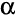# Repeated Measures Single Factor Experiment

A repeated measures single factor experiment is an experiment where both:

• One factor of two or more levels has been manipulated. For example, the experiment may be investigating the effect of different levels of price, or different flavors, or different advertisements. (Where two or more factors are manipulated, such as both price and flavor being varied, it is then a Multifactor Experiment and not a single factor experiment.)
• Each respondent in the survey has been shown all of the factors (e.g., if the experiment is comparing ten new products then each respondent rates all 10 of the products). (Incomplete block experiments, where respondents are only shown a subset of the levels, are analyzed in Q as Ranking Experiments and Multifactor Experiments).

## How to set up a repeated measures randomized single factor experiment in Q

1. Ensure that each level of the factor is represented by a separate variable. For example, if the experiment is testing the appeal of three different advertisements, A, B and C, then there should be three variables, one representing A, one representing B and one representing C. If the order with which the alternatives were shown to the respondents has been randomized, then this randomization needs to be removed, either at the time of creation of the data file, or, by creating new JavaScript Variables. (Alternatively, the experiment can be analyzed as a Ranking Experiment or a Multifactor Experiment, but this will generally be more complex than removing the randomization).
2. Set the Question Type to either:
3. Select the cells on the table and press### Example

This example uses a Pick One - Multi question in the Cola.sav project, which is by default installed on your computer at C:\Program Files\Q\Examples. A NET of WEEKLY+ has been created and its cells are selected. Thus, the repeated measures test is comparing the proportions of people to consumer each of these brands once a week or more. Particular aspects to note about the output are:

• An overall test is conducted. In this case it is Cochran's Q.
• Multiple comparisons have also been conducted between the categories. (Additionally, Column Comparisons can be added directly to the table.)

Please refer to Planned ANOVA-Type Tests for a description of how to interpret the outputs of this test. We would obtain the same test if instead selecting the cells of a Pick Any question.

This next example uses the means of the Pick One - Multi question. Again, we would get the same results if instead analyzing this question as a Number - Multi question.

## Non-parametric and limited dependent variable repeated measures models

When the question is set as a Number - Multi Q will, by default, model it as Repeated Measures ANOVA with Greenhouse & Geisser Epsilon Correction (i.e., a two-way ANOVA where the blocking variable is the first component). That is, the model tests for differences in the means of the data.

Changing the Statistical Assumptions setting of Means from t-test to Non-parametric will cause Q to instead conduct Friedman Test for Correlated Samples.

If the dependent variable is Ordered Categorical you can instead change the Question Type to Ranking and the test will look for differences in the relative order of preferences (see Ranking Experiments).

If the dependent variable is Categorical you can treat the data as a Multifactor Experiment and model using a multinomial logit